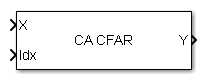Documentation

# CFAR Detector

Constant false alarm rate (CFAR) detector

## Library

Detection

`phaseddetectlib`## Description

The CA CFAR block implements a constant false-alarm rate detector using an estimate of the noise power. The CFAR detector estimates noise power from neighboring cells surrounding the cell under test. There are four methods for estimating noise: cell-averaging (CA), greatest-of cell averaging (GOCA), smallest-of cell averaging (SOCA), and order statistics (OS).

## Parameters

CFAR algorithm

Specify the CFAR detection algorithm using one of the values

 `CA` Cell-averaging `GOCA` Greatest-of cell averaging `OS` Order statistic `SOCA` Smallest-of cell averaging
Number of guard cells

Specify the number of guard cells used in training as an even integer. This parameter specifies the total number of cells on both sides of the cell under test.

Number of training cells

Specify the number of training cells used in training as an even integer. Whenever possible, the training cells are equally divided before and after the cell under test.

Rank of order statistic

This parameter appears when CFAR algorithm is set to `OS`. Specify the rank of the order statistic as a positive integer scalar. The value must be less than or equal to the value of Number of training cells.

Threshold factor method

Specify whether the threshold factor comes from an automatic calculation, the Custom threshold factor parameter, or an input argument. Values of this parameter are:

 `Auto` The application calculates the threshold factor automatically based on the desired probability of false alarm specified in the Probability of false alarm parameter. The calculation assumes each independent signal in the input is a single pulse coming out of a square law detector with no pulse integration. The calculation also assumes the noise is white Gaussian. `Custom` The Custom threshold factor parameter specifies the threshold factor. `Input port` Threshold factor is set using the input port `K`. This port appears only when Threshold factor method is set to `Input port`.
Probability of false alarm

This parameter appears only when you set Threshold factor method to `Auto`. Specify the desired probability of false alarm as a scalar between 0 and 1 (not inclusive).

Custom threshold factor

This parameter appears only when you set Threshold factor method to `Custom`. Specify the custom threshold factor as a positive scalar.

Output format

Format of detection results returned in output port `Y`, by the specified as `'CUT result'` or ```'Detection index'```.

• When set to `'CUT result'`, the results are logical detection values (`1` or `0`) for each tested cell. `1` indicates that the value of the tested cell exceeds a detection threshold.

• When set to `'Detection index'`, the results form a vector or matrix containing the indices of tested cells which exceed a detection threshold.

Output detection threshold

Select this check box to create an output port `Th` containing the detection threshold.

Output estimated noise power

Select this check box to create an output port `N` containing the estimated noise.

Source of the number of detections

Source of the number of detections, specified as `Auto` or `Property`. When you select `Auto`, the number of detection indices reported is the total number of cells under test that have detections. If you select `Property`, the number of reported detections is determined by the value of the Maximum number of detections parameter.

To enable this parameter, set the Output format parameter to `Detection index`.

Maximum number of detections

Maximum number of detection indices to report, specified as a positive integer.

To enable this parameter, set the Output format parameter to `Detection index` and the Source of the number of detections parameter to `Property`.

Simulate using

Block simulation method, specified as `Interpreted Execution` or ```Code Generation```. If you want your block to use the MATLAB® interpreter, choose `Interpreted Execution`. If you want your block to run as compiled code, choose `Code Generation`. Compiled code requires time to compile but usually runs faster.

Interpreted execution is useful when you are developing and tuning a model. The block runs the underlying System object™ in MATLAB. You can change and execute your model quickly. When you are satisfied with your results, you can then run the block using ```Code Generation```. Long simulations run faster than they would in interpreted execution. You can run repeated executions without recompiling. However, if you change any block parameters, then the block automatically recompiles before execution.

When setting this parameter, you must take into account the overall model simulation mode. The table shows how the Simulate using parameter interacts with the overall simulation mode.

When the Simulink® model is in `Accelerator` mode, the block mode specified using Simulate using overrides the simulation mode.

Acceleration Modes

 Block Simulation Simulation Behavior `Normal` `Accelerator` `Rapid Accelerator` `Interpreted Execution` The block executes using the MATLAB interpreter. The block executes using the MATLAB interpreter. Creates a standalone executable from the model. `Code Generation` The block is compiled. All blocks in the model are compiled.

## Ports

### Note

The block input and output ports correspond to the input and output parameters described in the `step` method of the underlying System object. See link at the bottom of this page.

PortDescriptionSupported Data Types
`X`

Input cell matrix.

The size of the first dimension of the input matrix can vary to simulate a changing signal length. A size change can occur, for example, in the case of a pulse waveform with variable pulse repetition frequency.

Double-precision floating point
`Idx`

Cells under test.

Double-precision floating point
`K`

Threshold factor.

Double-precision floating point
`N`

Noise power.

Double-precision floating point

`Y`

Detection results.

Double-precision floating point